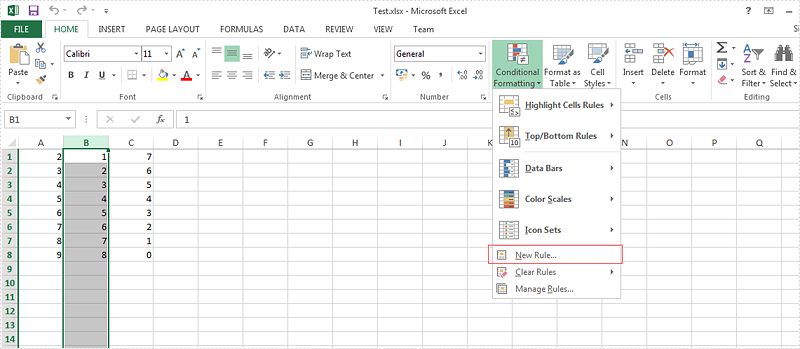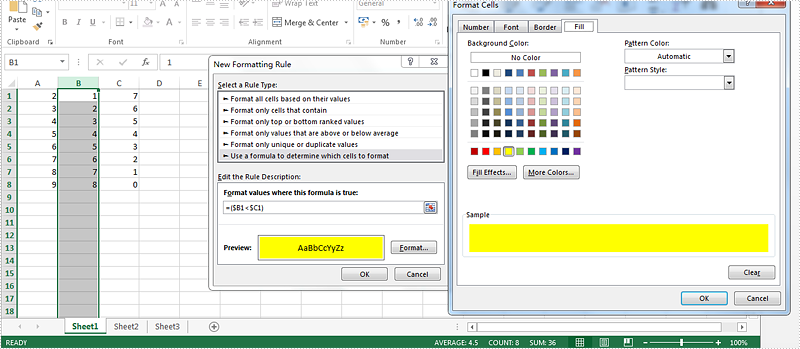Wednesday, 11 May 2016 07:40

## How to create a formula to apply conditional formatting in Excel in C#

Written by  support iceblue
Rate this item

We always use conditional formatting to highlight the cells with certain color from the whole data in the Excel worksheet. Spire.XLS also supports to create a formula to apply conditional formatting in Excel in C#. This article will show you how to apply a conditional formatting rule.

View the steps of Microsoft Excel set the conditional formatting.

Step 1: Choose the column B and then click "New Rule" under "Conditional Formatting"Step 2: Select the rule type, enter the rule by adding the formula and then add the highlight color for the format.Here comes to the steps of how to set the conditional formatting rule by Spire.XLS in C#.

Step 1: Create a new excel workbook and load the document from file.

```Workbook wb = new Workbook();
```

Step 2: Get the first worksheet and the second column from the workbook.

```Worksheet sheet = wb.Worksheets;
CellRange range = sheet.Columns;
```

Step 3: Set the conditional formatting formula and apply the rule to the chosen cell range.

```ConditionalFormatWrapper conditional = range.ConditionalFormats.AddCondition();
conditional.FormatType = ConditionalFormatType.Formula;
conditional.FirstFormula = "=(\$B1<\$C1)";
conditional.BackKnownColor = ExcelColors.Yellow;
```

Step 4: Save the document to file.

```wb.SaveToFile("result.xlsx", ExcelVersion.Version2010);
```

Effective screenshot:Full codes:

```static void Main(string[] args)
{
Workbook wb = new Workbook();

Worksheet sheet = wb.Worksheets;
CellRange range = sheet.Columns;

conditional.FormatType = ConditionalFormatType.Formula;
conditional.FirstFormula = "=(\$B1<\$C1)";
conditional.BackKnownColor = ExcelColors.Yellow;

wb.SaveToFile("result.xlsx", ExcelVersion.Version2010);

}
```